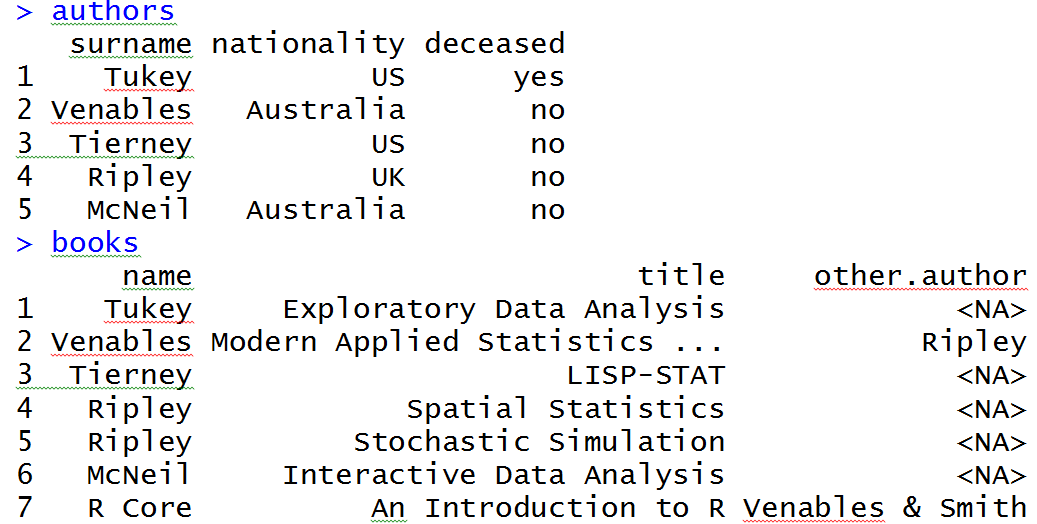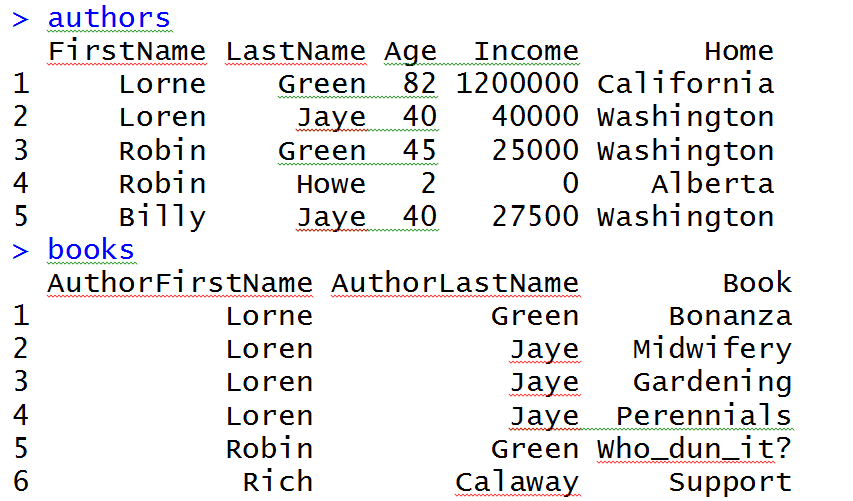# 首先看看排序：

sort(x)是对向量x进行排序，返回值排序后的数值向量。

rank()是求秩的函数，它的返回值是这个向量中对应元素的“排名”。

order()的返回值是对应“排名”的元素所在向量中的位置。

> x<-c(97,93,85,74,32,100,99,67)

> sort(x)

  32  67  74  85  93  97  99 100

> order(x)

 5 8 4 3 2 1 7 6

> rank(x)

 6 5 4 3 1 8 7 2

> x[order(x)]

  32  67  74  85  93  97  99 100

dat[order(dat[,1]),] 以该数据框的第一列进行排序

dat[order(dat[,1],dat[,2]),] 以该数据框的第一列为主要次序，第二列为次要序列进行排序

# 然后我们看看集合运算：

#首先对集合A,B,C赋值

> A<-1:10

> B<-seq(5,15,2)

> C<-1:5

> #求A和B的并集

> union(A,B)

  1  2  3  4  5  6  7  8  9 10 11 13 15

> #求A和B的交集

> intersect(A,B)

 5 7 9

> #求A-B

> setdiff(A,B)

  1  2  3  4  6  8 10

> #求B-A

> setdiff(B,A)

 11 13 15

> #检验集合A,B是否相同

> setequal(A,B)

 FALSE

> #检验元素12是否属于集合C

> is.element(12,C)

 FALSE

> #检验集合A是否包含C

> all(C%in%A)

 TRUE

> all(C%in%B)

>B

  5  7  9 11 13 15

>C

 1 2 3 4 5

>match(C,B)

 NA NA NA NA  1

>C%in%B

 FALSE FALSE FALSE FALSE  TRUE

# 接下来我们看看reshape：

# example of melt function
library(reshape)
mdata <- melt(mydata, id=c("id","time"))

 id time variable value 1 1 x1 5 1 2 x1 3 2 1 x1 6 2 2 x1 2 1 1 x2 6 1 2 x2 5 2 1 x2 1 2 2 x2 4

# cast the melted data
# cast(data, formula, function)
subjmeans <- cast(mdata, id~variable, mean)
timemeans <- cast(mdata, time~variable, mean)

subjmeans

 id x1 x2 1 4 5.5 2 4 2.5

timemeans

 time x1 x2 1 5.5 3.5 2 2.5 4.5

# 最后我们来看看merge函数：m1 <- merge(authors, books, by.x = "surname", by.y = "name")

m1 <- merge(authors, books, by.x = "surname", by.y = "name",all.x=TRUE)

right join功能代码

m1 <- merge(authors, books, by.x = "surname", by.y = "name",all.y=TRUE)

all join功能代码

m1 <- merge(authors, books, by.x = "surname", by.y = "name",all=TRUE)

x <- data.frame(k1 = c(NA,NA,3,4,5), k2 = c(1,NA,NA,4,5), data = 1:5)

y <- data.frame(k1 = c(NA,2,NA,4,5), k2 = c(NA,NA,3,4,5), data = 1:5)merge(authors, books, by=1:2)

merge(authors, books, by.x=c("FirstName", "LastName"),

by.y=c("AuthorFirstName", "AuthorLastName"),

all.x=TRUE)

A[with(A, paste(C1, C2, sep = "\r")) %in% with(B, paste(C1, C2, sep="\r")), ]

http://blog.sina.com.cn/s/blog_6caea8bf010159dt.html

http://blog.csdn.net/u014801157/article/details/24372441

http://www.statmethods.net/management/reshape.html

https://docs.tibco.com/pub/enterprise-runtime-for-R/1.5.0_may_2013/TERR_1.5.0_LanguageRef/base/merge.html

http://r.789695.n4.nabble.com/Matching-multiple-columns-in-a-data-frame-td890832.html